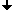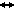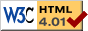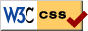﻿ Seminar Geschichte(n) der Dynamik 2, Winter 2020/2021
Nonlinear Dynamics at the Free University Berlin

Wintersemester 2020/2021

# Seminar Geschichte(n) der Dynamik 2

## Schedule

Wednesdays 10.00-12.00
No seminar on Wednesday 04.11.20! The distribution of topics will be on Thursday 05.11.20, at 12:00, after the lecture on Dynamical Systems 2.

## Beschreibung

In parallel to the lecture course "Dynamical Systems 2", we want to explore some exciting methods, examples and applications of dynamical systems, such as curiosities amongst discrete systems, fractals, oscillation patterns, and systems with time delays. We will chose a selection of the following topics, depending on the interests of the participants.

## Themen

### 1.1 Computer Simulations

• logistic map
• Hénon map
• Lorenz attractor
• Literature: [ASY96]

• iterates of unimodal maps
• Literature: [CE09]

### 1.3 The Hénon-Attraktor

• approximation of the logistic flow through Euler und central differences
• Hénon map as interpolation of both approximations
• period doubling in the Hénon map
• Computer experiments
• Literature: [HK12]

### 2.1 Fractals and non-integer dimensions

• What are fractals? Examples
• Hausdorff measure
• Hausdorff dimension with examples
• if time allows: compare to box-counting dimension
• Literature: [Fal04], notes

### 2.2 Self-similar fractals

• Examples: Cantor set, Sierpinski triangle, Koch curve
• Iterated function systems
• Literature: [Fal04], notes

### 2.3 Michael Crichton: the monster of Jurassic Park

• Construction of the dragon curve
• properties of the dragon curve
• Literature: [Cri90], exercise problem and notes

### 3.1 Inverse Pendulum

• Poincaré map, stability, swing, forced inverse pendulum
• If time allows: averaging, resonance
• Literature: [Arn92], [MW66]

### 3.2 The Kepler problem

• duality between Hooke’s law and the gravitational law
• Proof that orbits are elliptic
• Literature: [Arn90]

### 3.3 Hopf bifurcation

• The Stuart-Landau oscillator
• existence of bifurcating periodic solutions
• if time allows: stability
• Literature: [Kuz13]
• s

### 3.4 Romeo and Julia: Turing instability in love

• Stability / instability in coupled iterated systems
• Literature: [Fie10]

### 3.5 How the zebra got its stripes

• Reaction-diffusion equations
• Patterns in the Schnakenberg model
• Literature: [Mur88], notes

### 4.1 Introduction and methods of steps

• Examples for delay equations
• What is a solution? In which space does it live?
• method of steps, continuity of solutions
• Literature: [Hal77], [HL93], [Smi11], [Dri77]

### 4.2 Small solutions and solving backwards

• What is a small solution? Why can it occur?
• explicit examples
• Non-uniqueness of backwards solutions
• Literature: [Hal77], [HL93], [Smi11], [Dri77]

### 4.3 Stability and characteristic equations

• stability of solutions
• exponential ansatz and characteristic equations
• Literature: [Hal77; HL93; Smi11]

### 4.4 Nicholson’s blowflies equation

• Modeling of Nicholson’s blowflies equation
• Stability of non-trivial steady state
• Existence of periodic solutions
• Literature: [BBI10; GBN80]

## References

• [Arn90]   Vladimir I Arnold. Huygens and Barrow, Newton and Hooke: pioneers in mathematical analysis and catastrophe theory from evolvents to quasicrystals. Springer Science & Business Media, 1990.
• [Arn92]   Vladimir I. Arnold. Ordinary Differential Equations. Springer, 1992.
• [ASY96]   Kathleen T Alligood, Tim D Sauer, and James A Yorke. Chaos. Springer, 1996.
• [BBI10]   L Berezansky, Elena Braverman, and L Idels. “Nicholson’s blowflies differential equations revisited: main results and open problems”. In: Applied Mathematical Modelling 34.6 (2010), pp. 1405–1417.
• [CE09]   Pierre Collet and J-P Eckmann. Iterated maps on the interval as dynamical systems. Springer Science & Business Media, 2009.
• [Cri90]   Michael Crichton. Jurassic park: A novel. Vol. 1. Ballantine Books, 1990..
• [Dri77]   Rodney David Driver. Ordinary and delay differential equations. Vol. 20. Springer Science & Business Media, 1977.
• [Fal04]   Kenneth Falconer. Fractal geometry: mathematical foundations and applications. John Wiley & Sons, 2004.
• [Fie10]   Bernold Fiedler. “Romeo and Juliet, Spontaneous Pattern Formation, and Turing’s Instability”. In: Mathematics Everywhere (2010), p. 53.
• [GBN80]   WSC Gurney, SP Blythe, and RM Nisbet. “Nicholson’s blowflies revisited”. In: Nature 287.5777 (1980), pp. 17–21.
• [Hal77]   Jack K Hale. Theory of functional differential equations. Vol. 3. Springer, 1977.
• [HK12]   Jack K Hale and Hüseyin Koçak. Dynamics and bifurcations. Vol. 3. Springer Science & Business Media, 2012.
• [HL93]   Jack K Hale and Sjoerd M Verduyn Lunel. Introduction to functional differential equations. Vol. 99. Springer Science & Business Media, 1993.
• [Kuz13]   Yuri A Kuznetsov. Elements of applied bifurcation theory. Vol. 112. Springer Science & Business Media, 2013.
• [Mur88]   James D Murray. “How the leopard gets its spots”. In: Scientific American 258.3 (1988), pp. 80–87.
• [MW66]   Wilhelm Magnus and Stanley Winkler. Hill’s equation. Interscience, 1966.
• [Smi11]   Hal L Smith. An introduction to delay differential equations with applications to the life sciences. Vol. 57. Springer New York, 2011.
Further literature will either be provided on the webpage or communicated directly to the speakers.Last change: Oct. 26, 2020
 This page strictly conforms to the HTML4.01 standard and uses style sheets.